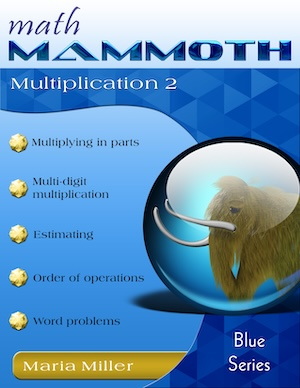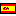^
You are here: HomeBlue Series → Multiplication 2

# Math Mammoth Multiplication 285 pages

Sample pages (PDF)

## Prices & ordering

Printed copy \$12.05

(credit cards, Google Pay, Paypal, PO)Este libro en español

Math Mammoth Multiplication 2 is a continuation from Math Mammoth Multiplication 1. It covers multi-digit multiplication and related topics, and suits best fourth grade mathematics.

The PDF version of this book is enabled for annotation. This means that if you prefer, your student can fill it in on the computer, using the typewriter and drawing tools in Adobe Reader version 9 or greater.

The first lessons briefly review the multiplication concept and the times tables. The next lesson, where students solve scales or pan balance problems, is intended to be somewhat fun and motivational. The balance problems are actually equations in disguise.

Then, the focus is on multi-digit multiplication (also called the algorithm of multiplication, or multiplying in columns). We start out by multiplying by whole tens and hundreds (such as 20 × 4 or 500 × 6). After this is mastered, we study a very important concept of multiplying in parts (also called partial products algorithm). It means that 4 × 63 is done in two parts: 4 × 60 and 4 × 3, and the results are added.

This principle underlies all other multiplication algorithms, so it is important to master it. We do not want children to learn the multiplication algorithm blindly, without understanding what is going on with it. Multiplying in parts is also tied in with an area model, which, again, is very important to understand.

Before showing the traditional form of multiplication, the lesson Multiply in Columns—the Easy Way shows a simplified form of the same, which is essentially just multiplying in parts. You may skip that lesson at your discretion or skim through it quickly if your child is ready to understand the standard form of the algorithm, which is taught next.

Students also study estimation, the order of operations, and multiplying with money. Many kinds of word problems abound in all of the lessons. Students are supposed to practice writing a number sentence for the word problems—essentially writing down the calculating they are doing.

The lesson So Many of the Same Thing could be entitled "Proportional Reasoning" but I wanted to avoid scaring parents and children with such a high-sounding phrase. The idea in that lesson is really simple, but it does prepare for proportions as they are taught in 7th grade and in algebra.

After that, we multiply two-digit numbers by two-digit numbers. Again, we first study partial products and tie that in with an area model. The lesson Multiplying in Parts: Another Way is optional. The books ends with the standard algorithm for multiplying a two-digit number by a two-digit number.

You might also be interested in:
• Math Mammoth Division 2—a worktext about long division, word problems, average, and divisibility (grades 4-5).
• Math Mammoth Multiplication & Division 3—a worktext about multi-digit multiplication and long division with larger numbers, ratios, simple equations, divisibility, primes, and factoring (grades 5-6).
• Make It Real Learning: Arithmetic I—an activity workbook with real-world data, focusing on situations that use addition, subtraction, estimation, division, bar graphs, and place value with large numbers (grades 3-6).

## Better Yet - Bundle Deals!

*BONUS*: Buy any bundle listed above, and get the fully upgraded Soft-Pak software for FREE (includes 4 math and 2 language arts programs, complete with on screen and printable options).

## How and where to order

You can buy Math Mammoth books at:

• Here at MathMammoth.com website — simply use the "Add to cart" buttons you see on the product pages.
• Rainbow Resource carries printed copies for the Light Blue series books, plus several CDs (Light Blue and Blue series).
• At Teachers Pay Teachers you can purchase the Light Blue Series downloads, plus topical units.
• Lulu sells printed copies for most of the Math Mammoth materials (various series).

By purchasing any of the books, permission IS granted for the teacher (or parent) to reproduce this material to be used with his/her students in a teaching situation; not for commercial resale. However, you are not permitted to share the material with another teacher.

In other words, you are permitted to make copies for the students/children you are teaching, but not for other teachers' usage.

Math Mammoth books are PDF files. You will need Adobe Reader to view them, including if you use a Mac or Linux. You can try other PDF viewers, but they seem to either omit or mess up some of the images.
WAIT!

Receive my monthly collection of math tips & resources directly in your inbox — and get a FREE Math Mammoth book!You can unsubscribe at any time.

### Math Mammoth Tour

Confused about the different options? Take a virtual email tour around Math Mammoth! You'll receive:

An initial email to download your GIFT of over 400 free worksheets and sample pages from my books. Six other "TOURSTOP" emails that explain the important things and commonly asked questions concerning Math Mammoth curriculum. (Find out the differences between all these different-colored series!)

This way, you'll have time to digest the information over one or two weeks, plus an opportunity to ask me personally about the curriculum.
A monthly collection of math teaching tips & Math Mammoth updates (unsubscribe any time)### "Mini" Math Teaching Course

This is a little "virtual" 2-week course, where you will receive emails on important topics on teaching math, including:

- How to help a student who is behind
- Troubles with word problems
- Teaching multiplication tables
- Why fractions are so difficult
- The value of mistakes
- Should you use timed tests
- And more!

A GIFT of over 400 free worksheets and sample pages from my books right in the very beginning.A monthly collection of math teaching tips & Math Mammoth updates (unsubscribe any time)
Enter your email to receive math teaching tips, resources, Math Mammoth news & sales, humor, and more! I tend to send out these tips about once monthly, near the beginning of the month, but occasionally you may hear from me twice per month (and sometimes less often).• A GIFT of over 400 free worksheets and sample pages from my books.